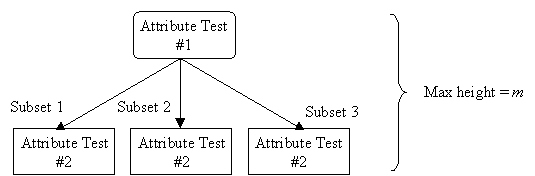References:

• T. Mitchell, "Decision Tree Learning", in T. Mitchell, Machine Learning, The McGraw-Hill Companies, Inc., 1997, pp. 52-78.
• P. Winston, "Learning by Building Identification Trees", in P. Winston, Artificial Intelligence, Addison-Wesley Publishing Company, 1992, pp. 423-442.

Robust to noisy data and capable of learning disjunctive expressions, decision tree learning, a method for approximating discrete-valued target functions, is one of the most widely used and practical methods for inductive inference.

Appropriate Problems for Decision Tree Learning

Decision tree learning is generally best suited to problems with the following characteristics:

• Instances are represented by attribute-value pairs.
• Instances are described by a fixed set of attributes (e.g., temperature) and their values (e.g., hot).
• The easiest situation for decision tree learning occurs when each attribute takes on a small number of disjoint possible values (e.g., hot, mild, cold).
• Extensions to the basic algorithm allow handling real-valued attributes as well (e.g., a floating point temperature).
• The target function has discrete output values.
• A decision tree assigns a classification to each example.
• Simplest case exists when there are only two possible classes (Boolean classification).
• Decision tree methods can also be easily extended to learning functions with more than two possible output values.
• A more substantial extension allows learning target functions with real-valued outputs, although the application of decision trees in this setting is less common.
• Disjunctive descriptions may be required.
• Decision trees naturally represent disjunctive expressions.
• The training data may contain errors.
• Decision tree learning methods are robust to errors - both errors in classifications of the training examples and errors in the attribute values that describe these examples.
• The training data may contain missing attribute values.
• Decision tree methods can be used even when some training examples have unknown values (e.g., humidity is known for only a fraction of the examples).

Learned functions are either represented by a decision tree or re-represented as sets of if-then rules to improve readability.

Decision Tree Representation

A decision tree is an arrangement of tests that prescribes an appropriate test at every step in an analysis.

In general, decision trees represent a disjunction of conjunctions of constraints on the attribute-values of instances. Each path from the tree root to a leaf corresponds to a conjunction of attribute tests, and the tree itself to a disjunction of these conjunctions.

More specifically, decision trees classify instances by sorting them down the tree from the root node to some leaf node, which provides the classification of the instance. Each node in the tree specifies a test of some attribute of the instance, and each branch descending from that node corresponds to one of the possible values for this attribute.

An instance is classified by starting at the root node of the decision tree, testing the attribute specified by this node, then moving down the tree branch corresponding to the value of the attribute. This process is then repeated at the node on this branch and so on untila leaf node is reached.

Diagram

• Each nonleaf node is connected to a test that splits its set of possible answers into subsets corresponding to different test results.
• Each branch carries a particular test result's subset to another node.
• Each node is connected to a set of possible answers.Occam's Razor (Specialized to Decision Trees)

"The world is inherently simple. Therefore the smallest decision tree that is consistent with the samples is the one that is most likely to identify unknown objects correctly."Given m attributes, a decision tree may have a maximum height of m.

Rather than building all the possible trees, measuring the size of each, and choosing the smallest tree that best fits the data, we use Quinlan's ID3 algorithm for constructing a decision tree.